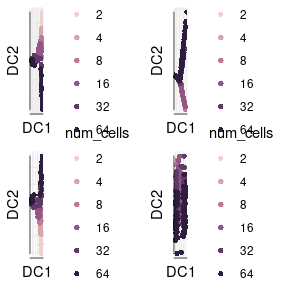# Gaussian kernel width sigma

The other important parameter for DiffusionMap is the Gaussian kernel width sigma ($$\sigma$$) that determines the transition probability between data points. The default call of destinyDiffusionMap(data) aka DiffusionMap(data, 'local')) – uses a local sigma per cell, derived from a local density estimate around each cell.

Using the 1.0 default, sigma = 'global', estimates sigma using a heuristic. It is also possible to specify this parameter manually to tweak the result. The eigenvector plot explained above will show a continuous decline instead of sharp drops if either the dataset is too big or the sigma is chosen too small.

The sigma estimation algorithm is explained in detail in Haghverdi, Buettner, and Theis (2015). In brief, it works by finding a maximum in the slope of the log-log plot of local density versus sigma.

library(destiny)
data(guo_norm)

## Using find_sigmas

An efficient variant of that procedure is provided by find_sigmas. This function determines the optimal sigma for a subset of the given data and provides the default sigma for a DiffusionMap call. Due to a different starting point, the resulting sigma is different from above:

sigmas <- find_sigmas(guo_norm, verbose = FALSE)
optimal_sigma(sigmas)
##  10.8946

The resulting diffusion map’s approximation depends on the chosen sigma. Note that the sigma estimation heuristic only finds local optima and even the global optimum of the heuristic might not be ideal for your data.

palette(cube_helix(6))

plots <- lapply(
list('local', 5, round(optimal_sigma(sigmas), 2), 100),
function(sigma) plot(
DiffusionMap(guo_norm, sigma), 1:2,
main = paste('σ =', sigma),
col_by = 'num_cells', draw_legend = FALSE))

do.call(gridExtra::grid.arrange, c(plots, ncol = 2))